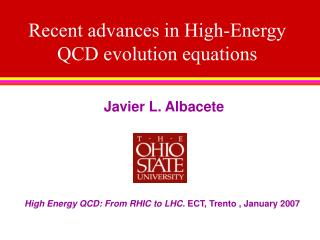DownloadDownload PresentationRecent advances in High-Energy QCD evolution equations

# Recent advances in High-Energy QCD evolution equations

Télécharger la présentation## Recent advances in High-Energy QCD evolution equations

- - - - - - - - - - - - - - - - - - - - - - - - - - - E N D - - - - - - - - - - - - - - - - - - - - - - - - - - -
##### Presentation Transcript

1. Recent advances in High-Energy QCD evolution equations Javier L. Albacete High Energy QCD: From RHIC to LHC. ECT, Trento , January 2007

2. INTRODUCTION B-JIMWLK provides the best theoretical tool so far for the description of high-energy scattering in QCD, but: • It is derived at Leading Logarithmic Accuracy (as ln1/x): Fixed coupling. Need of NLO calculation. • Valid for dense-dilute scattering: Symmetrization of the equations • It is derived in a mean field approach: Fluctuations, Pomeron loops… not taken into account Phenomenological consequences: • Reduction of the energy dependence of the saturation scale • Geometric scaling violations • More exclusive observables…

3. OUTLINE I: O(a2)-corrections to B-JIMWLK from the classical equations of motion. JHEP-0611:074, 2006, with N. Armesto and G. Milhano -The wave function formalism - Dipole limit - Diagrammatic interpretation II: Quark contribution to NLO-BK: some comments and numerical results. Heribert’s talk Y. Kovchegov and H. Weigert, hep-ph/0609090 I. Balitsky, hep-ph/0609105

4. O(a2)-corrections to JIMWLK from the classical equations of motion. DY r • B-JIMWLK can be derived using the ‘wave function formalism’ (projectile image) (Kovner, Lublinsky and Wiedemann) • In this approach the kernel of the evolution equation depends on the classical Weizsaker-Williams (WW) fields generated by the projectile charge density. • JIMWLK is obtained once the WW fields are taken at leading order in the projectile charge density. • We calculate the kernel when the projectile WW-fields are taken at second order in the projectile charge density. O( r): JIMWLK O(r2): This work

5. The wave function formalism Y WY Fundamental d.o.f. and c-number (high target density) • B-JIMWLK provides the HE evolution of a dense-dilute(target-projectile) system. • In the Light-ConeHamiltonian formalism and the LC gauge A+= 0 ‘valence’ gluons r ~ O(g)<< 1 eikonal, independent scattering Dense target : Wilson lines in the adjoint representation Averaging over target fields configurations:

6. z Y+dY WY The wave function formalism The evolution is managed boosting the projectile and leaving the target unevolved The boost opens up the phase space for new hard gluons emission Boosted wave function (valid only in the small projectile density limit!) Lorentz invariance

7. The wave function formalism The WW fields are determined by the classical Yang-Mills EOM r~O(1) Expansion of the WW-fields Expansion of the evol. kernel Leading order solution (independent gluon emission, ~ r ):

8. Second order solution in the projectile density (coherent, non-linear emission): • First correction to JIMWLK. Keeping terms up to O(g4): • Left and right rotation operators:

9. Dipole limit. Corrections to BK? Modified right and left rotation operators (ensure hermiticity of kernel): N-expansion:

10. Diagrammatic interpretation A mapping between the different terms in the kernels and diagrams can be done. (But this is a purely operational calculation!)

11. Diagrammatical interpretation of the kernel

12. LLL LRR

13. Final result: The leading 1/N correction vanishes: Using the symmetry of the wave function under the exchange of any number of dipoles (u,v) (r,p):

14. The corrections derived cannot be fully recasted in terms of dipoles degrees of freedom They include dipoles, quadrupoles, sextupoles, octupoles…

15. SUMMARY • There are no corrections to BK coming from higher order solutions of the projectile WW classical fields in the low projectile density limit. • The corrections to JIMWLK present a rather complicated color structure. They couple more terms to the Balitsky hierarchy

16. Some comments on the NLO quark contribution to small-x running coupling evolution Y. Kovchegov, H. Weigert, hep-ph/0609090 I. Balitsky, hep-ph/0609105

17. x x z1 z r1 r z2 r2 z y y • LO BK equation: • The complete NLO quark contribution to small-x non-linear evolution has been recently calculated. It contains running coupling and non-running coupling contributions:

18. x x z1 z1 z z z2 z2 y y • Thescheme dependence is due to the subtraction procedure: • Running coupling BK: • Balitsky • K-W

19. NUMERICAL RESULTS a 0.5 r INITIAL CONDITION MV: AN08: RUNNING COUPLING Landau Pole avoided freezing The coupling at large dipole size

20. AN08 init. cond

21. MV init. cond • The solutions obtained with Balitsky’s prescription propagate much slower than those obtained with KW’s. • The solutions obtained with KW’s prescription are much closer to those obtained with the running coupling scale set at the size of the parent dipole than those obtained with Balitsky’s

22. Geometric Scaling: The asymptotic scaling function is very litle scheme-dependente

23. Old work:

24. KW: Bal: Phenom: SATURATION SCALE WARNING: Just an example!! LHC MV init cond Fits to HERA data ?? Soyez’s talk

25. SATURATION SCALE WARNING: Just an example!! LHC AN08 Init cond KW: Bal: Phenom:

26. SUMMARY (Not so) Bad News • The new physical channels cannot be ignored: The solutions of BK equation with NLO quark contribution to the running coupling show a strong dependence on the scheme choice and on the initial condition. Strong phenomenological consequences: Good news: • Two independent and coincident calculations of the complete NLO quark contribution small-x evolution are available. • The subtraction piece is known and calculable (work in progress..). • This would us with a good theoretical extrapolation from RHIC to the LHC